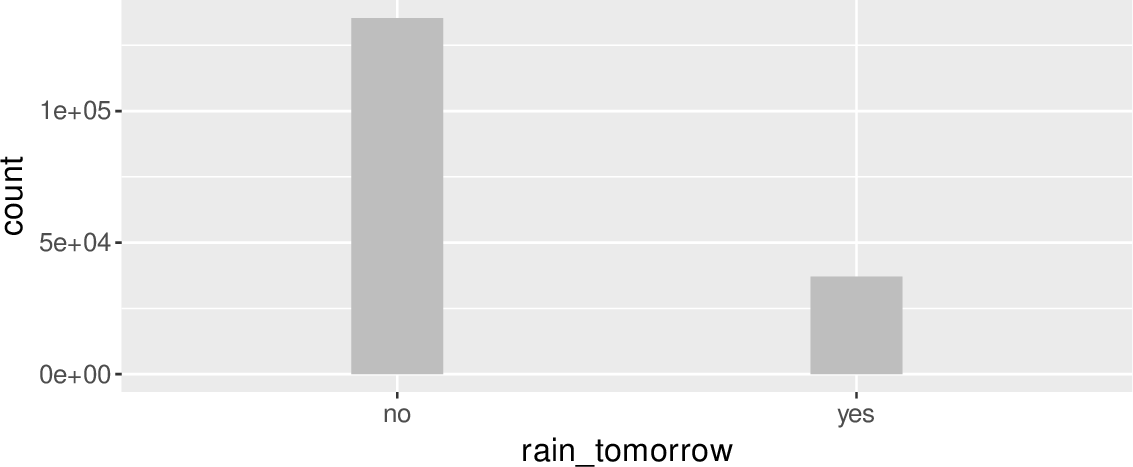Data Science Desktop Survival Guide by Graham WilliamsDesktop Survival Project Home Preface Data Science Introducing R R Constructs R Tasks R Strings R Read, Write, and Create Data Template Data Exploration Data Wrangling Data Visualisation Statistics ML Template ML Scenarios ML Activities ML Applications ML Algorithms Cluster Analysis Decision Trees Computer Vision Graph Data Privacy Literate Data Science Coding with Style Resources Bibliography Index

## Target as a Factor

20180726 We often build classification models. For such models we want to ensure the target is categoric. Often it is 0/1 and hence is loaded as numeric. We could tell our model algorithm of choice to explicitly do classification or else set the target using base::as.factor() in the formula. Nonetheless it is generally cleaner to do this here and note that this code has no effect if the target is already categoric.

# Ensure the target is categoric.

ds[[target]] %<>% as.factor()

# Confirm the distribution.

ds[target] %>% table()
 ```## . ## no yes ## 135353 37077 ```

We can visualise the distribution of the target variable using ggplot2. The dataset is piped to ggplot2::ggplot() whereby the target is associated through ggplot2::aes_string() (the aesthetics) with the x-axis of the plot. To this we add a graphics layer using ggplot2::geom_bar() to produce the bar chart, with bars having width= 0.2 and a fill= color of "grey". The resulting plot can be seen in Figure 9.1.

ds %>%
ggplot(aes_string(x=target)) +
geom_bar(width=0.2, fill="grey") +
theme(text=element_text(size=14))